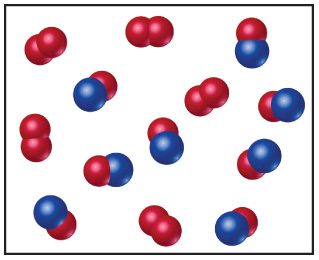# Problem: Nitrogen monoxide and oxygen react to form nitrogen dioxide. Consider the mixture of NO and O2 shown in the accompanying diagram. The blue spheres represent N, and the red ones represent O.If the actual yield of the reaction was 75% instead of 100%, how many molecules of NO2 would be present after the reaction was over?

###### FREE Expert Solution

We have to determine how many molecules of NO would be present in the reaction mixture after the reaction is complete.

Percent yield of a reaction can be calculated by this formula:

We will follow these steps to solve this problem:

Step 1: Write a balanced equation for the reaction.

Step 2: Determine the limiting reagent.

Step 3: Calculate the number of NO2 molecules at 75 % yield.###### Problem Details

Nitrogen monoxide and oxygen react to form nitrogen dioxide. Consider the mixture of NO and O2 shown in the accompanying diagram. The blue spheres represent N, and the red ones represent O.If the actual yield of the reaction was 75% instead of 100%, how many molecules of NO2 would be present after the reaction was over?